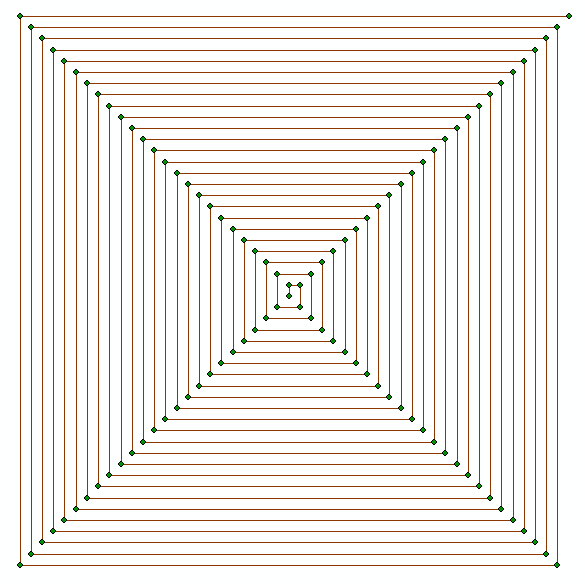# Creating an expanding square for search and rescue

1871
3
01-28-2016 08:00 AMOccasional Contributor

Tags (4)
3 RepliesbyMVP Honored Contributor

The example uses an approximation of geographic coordinates. It's kind of cool how they set up the even/odd routine. You can (perhaps) do better letting ArcGIS handle the coordinates through a projection (spatial reference).

In Python:

`>>> x = 0 # start x coord... y = 0 # start y coord... step = 10 # distance between lines... points = [] # points list container... line_array = arcpy.Array() # line vertices container... sr = arcpy.SpatialReference(3005) # spatial ref... for i in range(100): # we're going to make 100 points...    if i%2: # check even/odd...        if i/2%2: # check 1/2 even/odd...            x += step * int(i/2) # increase x...        else:...            x -= step * int(i/2) # decrease x...    else:...        if i/2%2: # check 1/2 even/odd...            y += step * int(i/2) # increase y...        else:...            y -= step * int(i/2) # decrease y...    point = arcpy.Point(x,y) # create point...    points.append(arcpy.PointGeometry(point,sr)) # add point to list...    line_array.add(point) # add location to line vertices... line = arcpy.Polyline(line_array,sr) # create line... arcpy.CopyFeatures_management(points, r'in_memory\points') # write points... arcpy.CopyFeatures_management(line, r'in_memory\line') # write line`Occasional Contributor

Wow, that was fast!  The code runs fine, but I'll have to play around with the spatial references. 3005 is for NAD_1983_BC_Environment_Albers so if you use latitude and longitude coordinates as the x,y input, it is not going to work.  What did you use as your inputs?byMVP Honored Contributor

The spatial reference is set in the line: sr = arcpy.SpatialReference(3005)

3005 is the factory code/authority code/EPSG code for BC Albers. Change it to the CRS of your choosing, but keep in mind that the units of 'step' will be determined by the CRS. If you input a geographic CRS, then the 'step' will be in units of degrees.